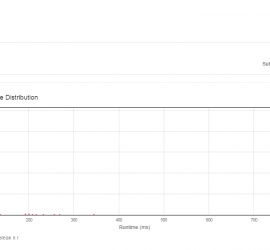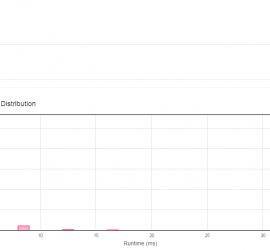# Monthly Archives: August 2015

## [leetcode] Binary Tree Postorder Traversal

Binary Tree Postorder Traversal Given a binary tree, return the postorder traversal of its nodes’ values. For example: Given binary tree {1,#,2,3}, 1 \ 2 / 3 return [3,2,1]. Note: Recursive solution is trivial, could you do it iteratively? Use two stacks to simulate recursion. stack st stores visiting nodes, […]## [leetcode] Reorder List

Reorder List Given a singly linked list L: L0→L1→…→Ln-1→Ln, reorder it to: L0→Ln→L1→Ln-1→L2→Ln-2→… You must do this in-place without altering the nodes’ values. For example, Given {1,2,3,4}, reorder it to {1,4,2,3}. The algorithm is straightforward. First, divide the linked list into two parts equally. (left part may be longer than […]## [leetcode] Word Break II

Word Break II Given a string s and a dictionary of words dict, add spaces in s to construct a sentence where each word is a valid dictionary word. Return all such possible sentences. For example, given s = “catsanddog”, dict = [“cat”, “cats”, “and”, “sand”, “dog”]. A solution is […]## [leetcode] Word Break

Word Break Given a string s and a dictionary of words dict, determine if s can be segmented into a space-separated sequence of one or more dictionary words. For example, given s = “leetcode”, dict = [“leet”, “code”]. Return true because “leetcode” can be segmented as “leet code”. First, I […]## [leetcode] Single Number II

Single Number II Given an array of integers, every element appears three times except for one. Find that single one. Note: Your algorithm should have a linear runtime complexity. Could you implement it without using extra memory? This is an extremely tricky problem. First, we consider each bit of the […]## [leetcode] Clone Graph

Clone an undirected graph. Each node in the graph contains a label and a list of its neighbors. OJ’s undirected graph serialization:Nodes are labeled uniquely.We use # as a separator for each node, and , as a separator for node label and each neighbor of the node.As an example, consider […]## [leetcode] Palindrome Partitioning II

Given a string s, partition s such that every substring of the partition is a palindrome. Return the minimum cuts needed for a palindrome partitioning of s. For example, given s = “aab”, Return 1 since the palindrome partitioning [“aa”,”b”] could be produced using 1 cut. Dynamic programming. dp[i] stores […]## [leetcode] Palindrome Partitioning

Given a string s, partition s such that every substring of the partition is a palindrome. Return all possible palindrome partitioning of s. For example, given s = “aab”, Return [ [“aa”,”b”], [“a”,”a”,”b”] ] Dynamic Programming. I used vector<vector<string>> dp[s.size()] as dynamic programming array. dp[i] stores all the possible palindrome […]## [leetcode] Surrounded Regions

Given a 2D board containing ‘X’ and ‘O’, capture all regions surrounded by ‘X’. A region is captured by flipping all ‘O’s into ‘X’s in that surrounded region. For example, X X X X X O O X X X O X X O X X After running your function, […]## [Leetcode] Sum Root to Leaf Numbers

Given a binary tree containing digits from 0-9 only, each root-to-leaf path could represent a number. An example is the root-to-leaf path 1->2->3 which represents the number 123. Find the total sum of all root-to-leaf numbers. For example, 1 / \ 2 3 The root-to-leaf path 1->2 represents the number […]